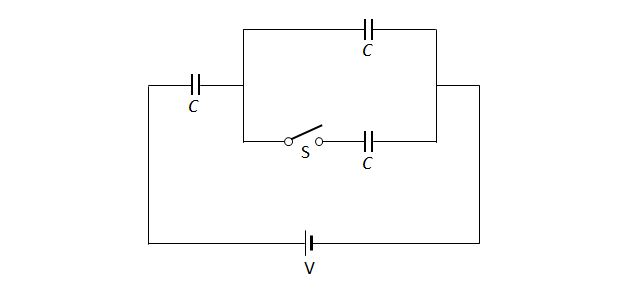# Three capacitorsThe above diagram is a circuit that consists of three capacitors with the same capacitance $C.$ When the switch is open, the quantity of the electric charge on the whole circuit is $Q.$ If the switch is closed, what will be the quantity of the electric charge on the whole circuit?

×